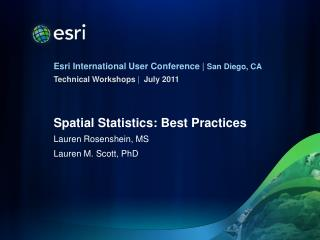DownloadDownload PresentationSpatial Statistics: Best Practices

# Spatial Statistics: Best Practices

Télécharger la présentation## Spatial Statistics: Best Practices

- - - - - - - - - - - - - - - - - - - - - - - - - - - E N D - - - - - - - - - - - - - - - - - - - - - - - - - - -
##### Presentation Transcript

1. July 2011 Spatial Statistics: Best Practices Lauren Rosenshein, MS Lauren M. Scott, PhD

2. Workshop Objectives • Demonstrate an analytical workflow, start to finish • 911 Emergency Call Data Analysis • Dig deeper: • Understand tool parameter options • Select an appropriate spatial scale for your analysis • Explore data relationships • Find a properly specified regression model • Point you to additional resources for learning more www.esriurl.com/spatialstats Kindly complete a course evaluation: www.esri.com/sessionevals

3. Context for the Analysis • Responding to 911 calls is expensive • The population is expected to double • This community has questions! • Are existing fire and police resources well sited? • What are the factors that contribute to high 911 call rates? • What can be done to reduce 911 call volumes? • Given population increases, what kinds of call volumes can we expect in the future?

4. Hot Spot Analysis • Works by assessing the values for each feature within the context of neighboring feature values • Challenges: • What is the analysis field? • With incident data, you often need to aggregate the data • What is the scale of your analysis? • Tools: • To aggregate your data • Integrate • Collect Events • To find an appropriate scale of analysis • Incremental Spatial Autocorrelation (sample script at 10.0) • Calculate Distance Band from Neighbor Count

5. Regression Analysis • All about answering “why?” questions • Ordinary Least Squares (OLS) creates an equation relating your dependent variable (calls) to a set of explanatory variables (population, income, …) • Challenge • Finding a “properly specified model” (a model you can trust …because it meets all of the assumptions of OLS) • Tool • Exploratory Regression

6. Did you find a good model? • Check coefficient significance and sign • You want explanatory variables that are good predictors • Each explanatory variable coefficient should: • Have the expected sign (the expected relationship) • Have an asterisk (*) indicating the variable is statistically significant • Check VIF values for Multicollinearity • You want a stable model: no explanatory variable redundancy • Variance Inflation Factor (VIF) values should be < 7.5 • Run the Spatial Autocorrelation tool on model residuals • You want to be able to trust variable relationships • Model over/under predictions should exhibit a random pattern

7. Did you find a good model? • Check the Jarque-Bera diagnostic for model bias • You want your model to be consistent for the full range of values and throughout the study area • Model over/under predictions should be normally distributed • Check model performance • You want a model that effectively explains 911 call volumes • Look for models with: • Largest Adjusted R2 value • Smallest AICc value